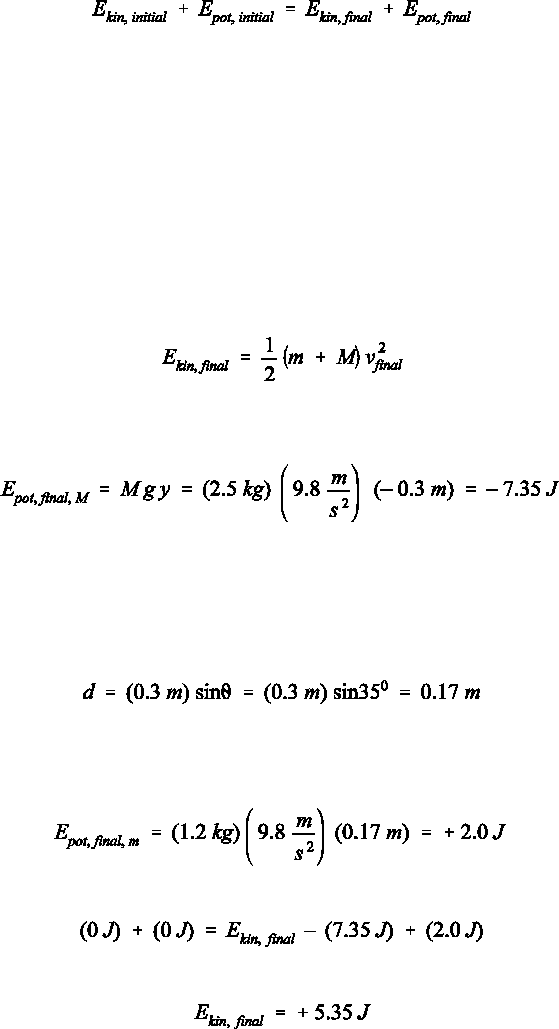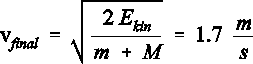# Physics 1028 Lecture 4: Chapter 7

3 views5 pages
School
Western University
Department
Physics
Course
Physics 1028
ProfessorSelection of Even–Numbered Problem Solutions
Chapter 7
P–7.12
We use the conservation of energy concept, written as:
(1)
for which we first identify the initial and final instants. Choose them such that the information available at
one instant is complete, and that the unknown parameter occurs at the other instant. It doesn't matter,
however, on which side of Eq.  the unknown parameter occurs.
We choose as the initial instant the moment of release when both objects are at rest. For the final
instant we choose the instant at which the object of mass M has fallen 30 cm since we are asked about the
total kinetic energy (unknown variable) at that point. Now we evaluate each term in Eq. :
! The initial kinetic energy is zero since both objects start from rest.
! The initial potential energy can be chosen arbitrarily since there is no predetermined origin along the
vertical axis which defines y = 0 in Epot = m g y for either object. For convenience, we choose y = 0 at the
initial height for each of the two objects and get Epot, init = 0 J. We also define the y–axis as directed upward.
! The final kinetic energy of the two objects is sought. We write this energy as Ekin, final = ½(m + M) v²final.
(2)
! The final potential energy has two contributions. The object of mass M is at this time at y = – 0.3 m, i.e.,
the contribution of this object to the final potential energy is:
(3)
This value is negative since the object is closer to Earth, which is associated with a decrease in the potential
energy. At the same time, the object of mass m has moved 30 cm up the inclined plane. We know this because
the massless string is taut, and therefore, does not change its length during the process. Since the object of
mass m moves along the inclined plane and not vertically up, its position relative to the surface of Earth has
not changed by a length of 0.3 m but by a distance d:
(4)
which is the y–component of the total displacement. Remember, the potential energy is a function of the
position relative to the surface of Earth; thus, only the change in the vertical position matters. From this we
now calculate the final potential energy of the object of mass m:
(5)
Next we enter all four terms we found into Eq. :
(6)
which yields:
(7)
The final kinetic energy is larger than zero since both objects are in motion when the object of mass M passes
the 30 cm marker. The result in Eq.  allows us to calculate the final speed:
Unlock document

This preview shows pages 1-2 of the document.
Unlock all 5 pages and 3 million more documents.(8)
The sum (m + M) occurs in the denominator because the objects are linked by the string, and therefore move
with the same speed at every instant.
Unlock document

This preview shows pages 1-2 of the document.
Unlock all 5 pages and 3 million more documents.

## Document Summary

We use the conservation of energy concept, written as: (1) for which we first identify the initial and final instants. Choose them such that the information available at one instant is complete, and that the unknown parameter occurs at the other instant. It doesn"t matter, however, on which side of eq. We choose as the initial instant the moment of release when both objects are at rest. For the final instant we choose the instant at which the object of mass m has fallen 30 cm since we are asked about the total kinetic energy (unknown variable) at that point. The initial kinetic energy is zero since both objects start from rest. The initial potential energy can be chosen arbitrarily since there is no predetermined origin along the vertical axis which defines y = 0 in epot = m g y for either object.